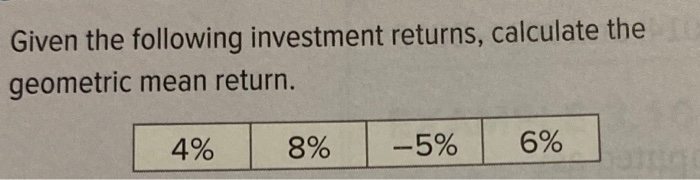# Question Given the following investment returns, calculate the geometric mean return. 4% 8% -5% 6%OHLO53 The Asker · Probability and StatisticsTranscribed Image Text: Given the following investment returns, calculate the geometric mean return. 4% 8% -5% 6%
More
Transcribed Image Text: Given the following investment returns, calculate the geometric mean return. 4% 8% -5% 6%